```

```
Wholeness and the Implicate Order
```
```
The Crystallization Process and the Implicate Order
(Part Twelve)

```

```
We  continue our investigation concerning the generation of group elements of the Plane Groups.
```
```
The Plane Group P3m1

Two distinct Plane Groups, P3m1 and P31m, belong to Point Group 3m. They have the same total symmetry content and shape, but the motifs differ in orientation with respect to the edges of the unit mesh. Let's start with Plane Group P3m1.

Placing motifs into a hexagonal net, but now motifs, having a point symmetry 3m -- meaning that each motif has a 3-fold rotation axis going through its center (and perpendicular to the plane of the drawing), and three equivalent mirror lines, such that their point of intersection coincides with the center of such a motif -- and oriented in the net such that their mirror lines do not coincide with the connecting lines of the net, yields a pattern (of repeated motifs) representing Plane Group P3m1. See Figure 1.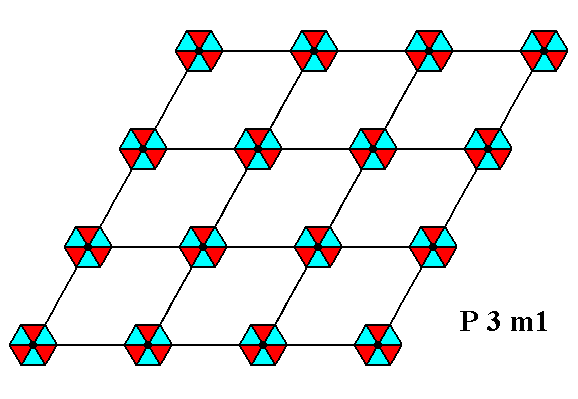Figure 1.  The insertion of motifs having 3m symmetry and oriented as described above, into a hexagonal net, leads to a periodic pattern representing Plane Group P3m1.
The symmetry of the motifs is indicated by their shape and by their coloration.

```
```Figure 2.  A unit mesh is chosen (yellow). It is primitive, and its point symmetry is m , meaning that its only symmetry element is a mirror line.

```
```
If the pattern representing Plane Group P3m1 is contracted such that all translations are eliminated, then we end up with a figure that has 3m symmetry. It is the translation-free residue of that Plane Group, and as such represents the Point Group to which that Plane Group belongs. See Figure 3.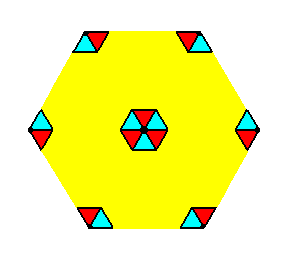Figure 3.  The translation-free residue of the Plane Group P3m1.
The symmetry of this residue is
3m, i.e. it has a 3-fold rotation axis going through its center and perpendicular to the plane of the drawing, and three equivalent mirror lines.

```
```
The translation-free residue is, in the present case, also the motif s.l., which means that as such it tiles the 2-D plane completely, just like the translation-free residue of a pattern representing the later to be treated Plane Group P6mm (See (click) HERE).

The symmetries involved in a pattern representing Plane Group P3m1 are 3-fold rotation axes, mirror lines and glide lines. See Figures 4, 5, 6 and 7.Figure 4.  A pattern representing Plane Group P3m1 has mirror lines. One of them is indicated.

```
```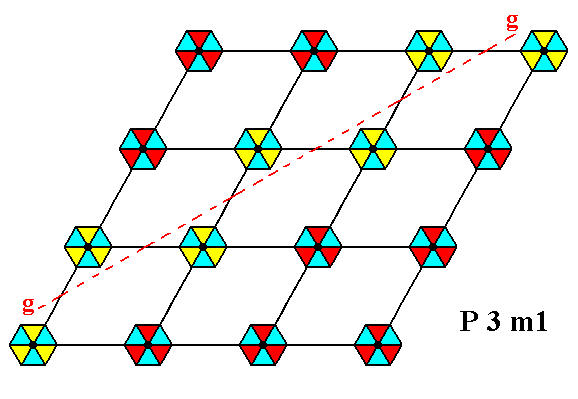Figure 5.  A pattern representing Plane Group P3m1 has glide lines. One of them is indicated.

```
```Figure 6.  A pattern representing Plane Group P3m1 has 3-fold rotation axes perpendicular to the plane of the drawing. One of them is indicated.

```
```Figure 7.  A pattern representing Plane Group P3m1 has 3-fold rotation axes perpendicular to the plane of the drawing. Again one of them is indicated (small blue triangle).

```
```
The total symmetry content of the Plane Group P3m1 is depicted in the next Figure.Figure 8.  Total symmetry content of the Plane Group P3m1.
3-fold axes are indicated by small solid blue triangles.
Glide lines are indicated by dashed red lines.
Mirror lines are indicated by solid red lines.

```
```
For clarity we depict this same total symmetry content, but now referring only to one mesh of the net :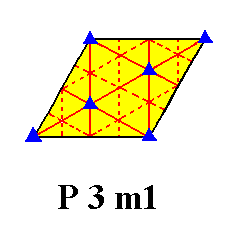Figure 9.  Total symmetry content of Plane Group P3m1, depicted for one mesh of the net. Only the red solid lines are mirror lines.

```

```
The generation of the group elements of the Plane Group P3m1

Each motif in Figure 10 is subdivided into three units. Each individual unit is still symmetric, and is, consequently not a basic motif unit. And only a basic motif unit can represent a group element, in our case an element of the group P3m1. The units as depicted in Figure 10 (i.e. augmented motif units) represent subgroup and cosets of the group P3m1 (For these latter concepts see Group Theory at our Second (Part of) Website, they are not of direct importance in the present context).Figure 10.  The insertion of motifs having 3m symmetry and oriented as described above, into a hexagonal net, leads to a periodic pattern representing Plane Group P3m1.
Each (composed) motif consists of three augmented motif units, such that the symmetry of the composed motif is
3m .  Each augmented motif unit can represent a subgroup or coset of the full group.
The pattern must be conceived as extending indefinitely in two-dimensional space.

```
```
As has been said, the motif units as depicted in Figure 10 are augmented motif units. To indicate the true basic motif units, representing group elements, we should indicate the composed nature of the augmented motif units. We can do that by inserting extra lines :or perhaps still better (i.e. a little more indicative) :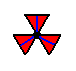And to further highlight the basic motif units that legitimately represent group elements, we could use colors, provided we do not interpret a difference of color as an asymmetry. The two (differently colored) parts of an augmented motif unit represent different group elements, but are nevertheless symmetrically related to each other :So now we have six basic motif units making up one composed motif of the P3m1 pattern.
To generate the full group we need one such basic motif unit to represent the identity element  1 ,  another basic motif unit to represent a first (of the three needed) generator, namely an anticlockwise rotation  p  of 1200 about a certain fixed lattice point (which we will call the point R),  yet another such unit to represent a second generator, namely a reflection in the line  m  making an angle of 300 with the horizontal lattice connection lines, and passing through the point R,  and, finally, yet another basic motif unit to represent the third generator, namely the translation  t .
Let's indicate the identity element, the rotation (first generator) and the reflection (second generator) in an enlarged composed motif :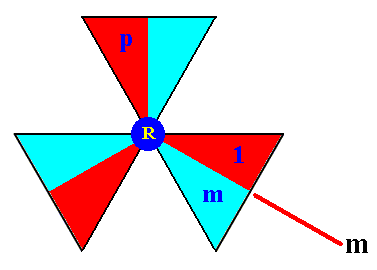Figure 11.  The composed motif at a chosen lattice point  R . As such it consists of six basic motif units representing elements of the group P3m1. One unit is chosen to be the identity element 1 , the other two are the generators  p  and  m .

```
```
Let us now put all this in the context of the lattice. We then indicate the lattice points R and S,  the identity element  1 ,  the generators  p  and  m ,  and the generator  t ,  which is a horizontal translation. See next Figure.Figure 12.  Tri-radiate composed motifs are inserted into a hexagonal point lattice, such that the three mirror lines of those motifs do not coincide with the lattice lines (i.e. with the edges of the rhomb-shaped unit cell, as indicated in the Figure), resulting in a periodic pattern according to the Plane Group P3m1.
Each composed motif consists of three augmented motif units, while each augmented motif unit consists of two basic motif units (red and blue) representing elements of the group P3m1.
One such basic motif unit (of the composed motif at the lattice point R ) is chosen to represent the identity element
1 .  A second basic motif unit is chosen to be the generator  p ,   a third one is chosen to be the generator  m ,  and a fourth basic motif unit (which is part of the composed motif at the point S ) is chosen to be the generator  t .
One mirror line, the line
m ,  is indicated. It is the symmetry element of the generator  m .

```
```
The identity of the remaining basic motif units of the composed motif at the lattice point R can now be determined ( p3 = 1 ) :Figure 13.  The group elements of the composed motif at the point R .
Together they form the subgroup
{1,  p,  p2 , m,  mp,  mp2} , having the structure of D3 .  The elements  1,  p,  p2  form a subgroup with the structure of C3 .  The elements  1  and  m  form the subgroup  C2 .

```
```
We will now produce the composed motif at the point S (Figure 12). We'll do this by subjecting all the elements (basic motif units) of the composed motif at the point R to a translation  t ,  i.e. we will form the left coset of the subgroup D3 (materialized as the composed motif at the point R) by the element  t . See next Figure.Figure 14.  The elements (basic motif units) of the composed motif at the lattice point S .  They form the left coset of the D3 subgroup by the element  t .  The notations (i.e. identities) of the newly generated elements are placed at the perimeter of the image (i.e. outside the image).

```
```
Next we determine the elements of the composed motif at the lattice point U  (See Figure 12).
To generate those elements we must subject the elements of the composed motif at the point S to an anticlockwise rotation of 2400 about the point R, that is to say, we must apply the transformation  p2 . In this way we form the left coset of the subgroup D3 by the element  p2t . See next Figure.Figure 15.  Generation of the basic motif units of the composed motif at the lattice point  U . The names of the newly generated elements are given at the perimeter of the image (i.e. outside the image).

```
```
The second and third row of composed motifs (Figure 12) can now be completed by applying the translations  . . . t-2, t-1, t,  t2, t3, . . . etc.
We will do so with respect to the composed motif at the point X (Figure 12) :Figure 16.  Generation of the elements of the composed motif at the point  X . Together they form the left coset of the subgroup  D3  by the element  t2 .
The notation of the newly generated elements is given at the perimeter of the image.

```
```
From this lastly obtained composed motif we can reach the fourth row (Figure 12) of composed motifs (to be generated), by applying  p2  to that lastly obtained composed motif. See next Figure.Figure 17.  Generation of the elements of the composed motif at the lattice point  W . Together they form the left coset of the subgroup  D3  by the element  p2t2 .
The notation of the newly generated elements is given at the perimeter of the image.

```
```
The fourth row can now be completed by translations. Continuation of this procedure will generate in principle the whole group  P3m1 , "in principle", because the order of the group is infinite.
```

```
Next we will generate this same group P3m1 by means of augmented motif units (instead of basic motif units as we just have done).
Such an augmented motif unit consists of two symmetrically related basic motif units. When using such augmented motif units a full composed motif will consist of three such motif units, and when we use the same notation as we did above, the composed motif at the lattice point R will look as follows :orfor that matter.
Because we now use augmented motif units, which themselves are symmetric, i.e. possess a mirror line, we do not need the generator  m  (i.e. an element resulting from a reflection of the initial element in a mirror line  m ).  The only generators we now need are  p ,  which is an anticlockwise rotation of 1200 about the point R ( This generator is -- as augmented motif unit -- indicated in the above two images of the composed motif at the point R),  and a (horizontal) translation  t ,  represented by an augmented motif unit  t . Also with respect to the augmented motif units,  p3 = 1  holds. The augmented motif units are based on the subgroup {1, m} consisting of the basic motif units  1  and  m .
Now our newly conceived motif units, the augmented motif units (consisting of two symmetrically related basic motif units), will be indicated by the same notation as was done above. The group P3m1 will then be generated by the augmented motif units  p  and  t .

The next Figure shows the two chosen generators :
The 'element'  p ,  represented by the augmented motif unit  p ,  that results from the element (augmented motif unit)  1  (chosen to be the identity element, initial motif unit), by an anticlockwise rotation of 1200 about the point  R .
The 'element'  t ,  that results from the 'element'  1  by a translation  t .Figure 18.  Two chosen generators  p  and  t  that can generate the whole  P3m1  pattern.

```
```Figure 19.  The whole  P3m1  pattern can be produced by the generator elements  p  and  t .

```

```
We will now again consider the generation of the group elements of our group  P3m1 ,  but now letting the group elements be represented by not only a basic unit of the motif s.str.  but also its maximal surroundings. This means that we must find areas of equal size and eaqual shape (but not necessarily of equal orientation) that completely tile our P3m1 pattern. To accomplish this we must first determine an appropriate choice of the motif s.l., which periodically tesselates the pattern, which means that the areas representing such motifs must not only be of equal size and shape, but also of equal orientation. See next Figure.Figure 20.  Motifs  s.l.  (yellow hexagons) of the  P3m1  pattern of Figure 12.  Each motif  s.str.  consists of six basic motif units (represented in the colors red and blue) which can represent group elements, but are now going to do so together with their proper surroundings (background). See Figures 22 and 23.

```
```
The next Figure gives one such motif s.l. isolated.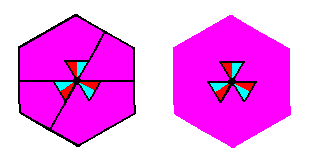Figure 21.  A motif  s.l.  of the  P3m1  pattern of Figure 12 isolated (background indicated by purple coloring).
Left image :  motif s.l. with lattice lines and other auxiliary lines.
Right image :  motif s.l. without such lines.

```
```
Now we will partition those motifs s.l. in such a way that each resulting area contains one basic unit of the motif s.str. PLUS its proper background, and moreover in such a way that those areas are equal in size and shape (but not necessarily equal in orientation), and tesselate the plane completely :Figure 22.  Partition of a motif  s.l.  into six areas  A, B, C, D, E, F ,  each representing a group element (and each containing one basic unit of the motif  s.str. ).

```
```Figure 23.  Partition of the motifs s.l. and with it of the  P3m1  pattern, such that the resulting areas (yellow and green triangles) represent group elements (  The colors yellow and green do not signify qualitative differences). Lattice lines are still indicated.

```
```
In this partitioned pattern we will now indicate the group element  1  (initial element, identity element), and the three generator elements  p, m  and  t ., where  p  represents an anticlockwise rotation of 1200 about the point  R ,  m  a reflection in the line  m ,  and  t  a horizontal translation. See next Figure.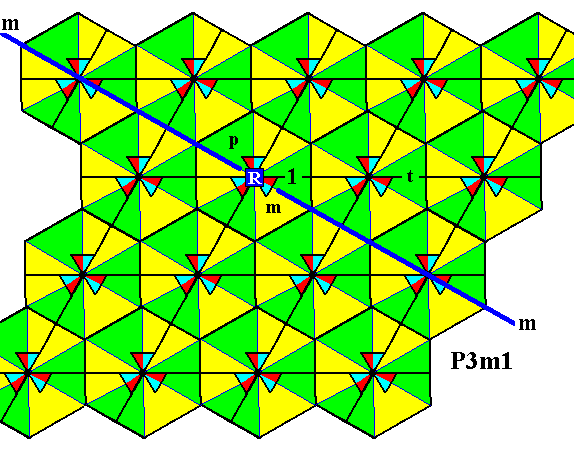Figure 24.  In the partitioned  P3m1  pattern four group elements (represented by triangular areas) are explicitly indicated :
The initial element
1 .
The generator
p .
The generator
m .
The generator
t .

```

```
In the next document we will generate group elements of our Group P3m1 by applying the generators.

e-mail :```
```
To continue click HERE for further study of the totally dynamic and holistic nature of Reality.
```
```
back to homepage

****************